Next: Bypassing the Bayes' theorem Up: Uncertainty due to systematic Previous: Indirect calibration   Contents

## Counting measurements in the presence of background

As an example of a different kind of systematic effect, let us think of counting experiments in the presence of background. For example we are searching for a new particle, we make some selection cuts and countevents. But we also expect an average number of background events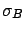, where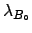is the standard uncertainty of, not to be confused with. What can we say about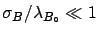, the true value of the average number associated with the signal? First we will treat the case in which the determination of the expected number of background events is well known (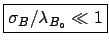), and then the general case.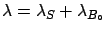:
The true value of the sum of signal and background is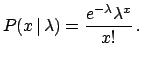. The likelihood is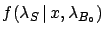(5.87)

Applying Bayes' theorem we have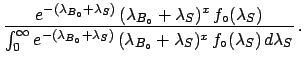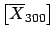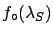(5.88)

Choosing again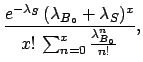uniform (in a reasonable interval) this gets simplified. The integral in the denominator can be calculated easily by parts and the final result is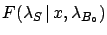(5.89)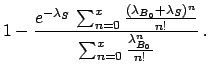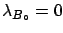(5.90)

From ()-() it is possible to calculate in the usual way the best estimate and the credibility intervals of. Two particular cases are of interest: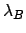:
In the general case, the true value of the average number of background events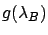is unknown. We only known that it is distributed aroundwith standard deviationand probability density function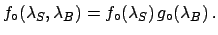, not necessarily a Gaussian. What changes with respect to the previous case is the initial distribution, now a joint function ofand of. Assumingandindependent the prior density function is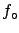(5.92)

We leave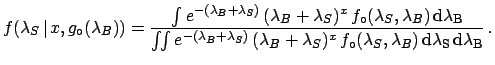in the form of a joint distribution to indicate that the result we shall get is the most general for this kind of problem. The likelihood, on the other hand, remains the same as in the previous example. The inference ofis done in the usual way, applying Bayes' theorem and marginalizing with respect to: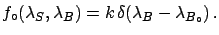(5.93)

The previous case [formula ()] is recovered if the only value allowed forisandis uniform:(5.94)Next: Bypassing the Bayes' theorem Up: Uncertainty due to systematic Previous: Indirect calibration   Contents
Giulio D'Agostini 2003-05-15